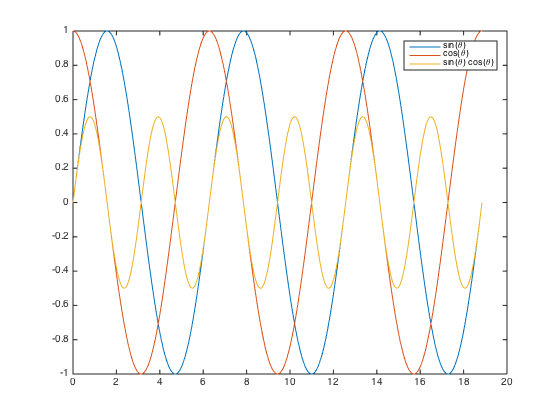# Euler’s formula and trig identities

I'm terrible at rote memorization.

I thought about that recently when I happened to see a plot (I think it was in a documentation example) that compared $sin(\theta)$, $cos(\theta)$, and $sin(\theta) cos(\theta)$.

theta = linspace(0,6*pi,500);
plot(theta,sin(theta))
hold on
plot(theta,cos(theta))
plot(theta,sin(theta) .* cos(theta))
hold off
legend({'sin(\theta)','cos(\theta)','sin(\theta) cos(\theta)'})Hmm, I thought. I guess $sin(\theta) cos(\theta)$ is just a sinusoid with twice the frequency and half the amplitude.

How is that related to memorization, you might ask? Well, I always had a very hard time remembering trig identities in high school and college. But, as an electrical engineering student, I had the following formula engraved on my brain cells:

$$e^{j\theta} = cos(\theta) + j sin(\theta)$$

(Note that I am following the proud electrical engineering tradition of using j instead of i as the imaginary unit. Because i obviously stands for current.)

This is called Euler's formula, and it's used all over the place in electrical engineering. I did not know at the time that Richard Feynman called it "the most remarkable formula in mathematics."

Here are a couple of closely related formulas:

$$cos(\theta) = \frac{1}{2} (e^{j\theta} + e^{-j\theta})$$

$$sin(\theta) = \frac{1}{2j} (e^{j\theta} - e^{-j\theta})$$

At some point during my undergraduate days, it dawned on me that I could derive many of the common trig identities directly from these formulas. Here's how it goes for $sin(\theta) cos(\theta)$:

$$sin(\theta) cos(\theta) = \frac{1}{2j} (e^{j\theta} - e^{-j\theta}) \frac{1}{2} (e^{j\theta} + e^{-j\theta})$$

$$sin(\theta) cos(\theta) = \frac{1}{4j} (e^{j2\theta} - e^{-j2\theta} + 1 - 1)$$

$$sin(\theta) cos(\theta) = \frac{1}{2} \frac{1}{2j} (e^{j2\theta} - e^{-j2\theta})$$

$$sin(\theta) cos(\theta) = \frac{1}{2} sin(2\theta)$$

Presto! Like magic.

I used to think that I must be kind of weird to prefer this manipulation of Euler's formula to simply memorizing the trig identities. But while writing this blog today, I noticed that the Wikipedia article on Euler's formula describes this concept exactly. "Complex exponentials can simplify trigonometry, because they are easier to manipulate than their sinusoidal components."

OK!

PS. In my plot creation code above, I exploited a subtle improvement in MATLAB R2014b graphics that customers have been requesting for many years. Can you spot it?

Published with MATLAB® R2014b

|